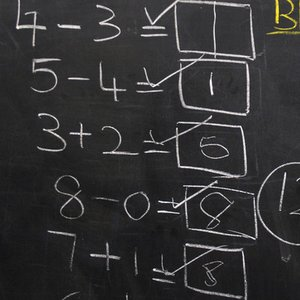# Top 10 math­e­mat­ics facts

Selected as the most interesting among mathematics related questions in
Augustus was born on 1 January 10BC. Which birthday did he celebrate at 1 January 10AD?
19. There was no year number zero. There were only 1999 years between 1000BC and 1000AD.
Correct this question
0
Correct this question
5
Very interesting!
What is six to the tenth power?
60466176. 6x6=36. And if so, then a further multiplication by six must leave the sixth in the last position. The subsequent powers of the six are 6, 36, 216, 1296, 7776, 46656 ...
Correct this question
0
Correct this question
4
Very interesting!
What is the sum of all even numbers between 1 and 100?
5050. 1+2+...+99+100 = 1+100+2+99+...+50+51 = 101+101+101+...+101 = 101*50 = 5050 It's a calculation method invented by Gauss.
Correct this question
0
Correct this question
4
Very interesting!
Play and see more questions